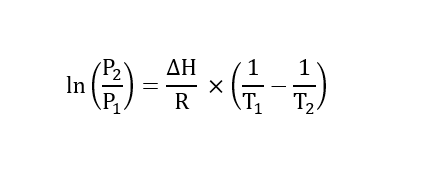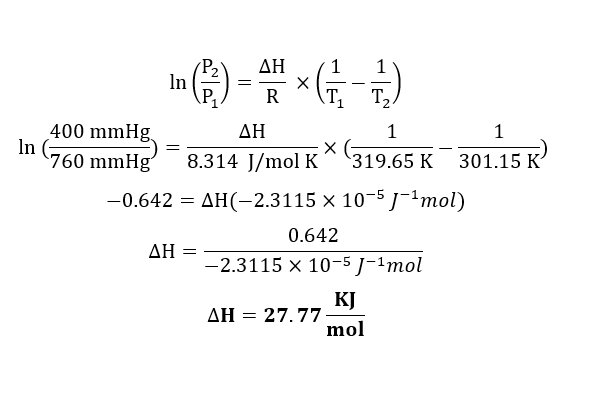# Carbon disulfide, CS2, is a volatile, flammable liquid. It has a vapor pressure of 400.0 mmHg at 28.0  degrees celsius and a normal boiling point of 46.5 degrees celsius.A) What is the heat of  vaporization of this substance in KJ/mol?B)At which pressure would CS2 have a boilingpoint of 75 degrees celsius?

Question
13 views

Carbon disulfide, CS2, is a volatile, flammable liquid. It has a vapor pressure of 400.0 mmHg at 28.0  degrees celsius and a normal boiling point of 46.5 degrees celsius.

A) What is the heat of  vaporization of this substance in KJ/mol?

B)At which pressure would CS2 have a boilingpoint of 75 degrees celsius?

check_circle

star
star
star
star
star
1 Rating
Given data:

Vapor pressure (P1) = 760 mmHg (normal boiling point pressure)

Normal boiling temperature (T1) = 46.5 ℃ = 319.65 K.

Vapor pressure (P2) = 400 mmHg

Final temperature (T2) = 28 ℃ = 301.15 K

Temperature (T3) = 75 ℃ = 348.15 K.

Formula:

Clausius-Clapeyron Equation is given by,A. Calculation for heat of vaporization using Clausius-Clapeyron Equation:...

### Want to see the full answer?

See Solution

#### Want to see this answer and more?

Solutions are written by subject experts who are available 24/7. Questions are typically answered within 1 hour.*

See Solution
*Response times may vary by subject and question.
Tagged in

### Chemistry